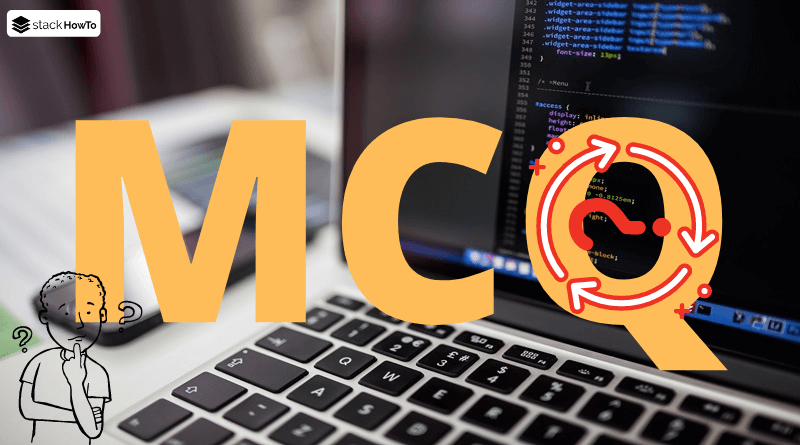MCQ

# Java MCQ – Multiple Choice Questions and Answers – Data Types and Variables – Part 2

This collection of Java Multiple Choice Questions and Answers (MCQs): Quizzes & Practice Tests with Answer focuses on “Java data types and variables”.

###### 1. In Java int, short, byte and long all of these are _________

A unsigned

B signed

C Both of the above

D None of these

B
In Java int, short, byte and long all of these are signed. Only “String” is unsigned, signed data means the data should be declared before using it.

###### 2. Which of the following is a valid declaration of a boolean?

A `boolean b1 = 'true';`

B `boolean b2 = false;`

C `boolean b3 = 'false';`

D `boolean b4 = 1;`

B
Boolean can only be assigned true or false literals.

###### 3. An expression include byte, int, and numbers is moved up to which of these?

A int

B long

C byte

D float

A
An expression include byte, int, and numbers is moved up to int before any calculation is done.

###### 4. What is the default value of a variable declared boolean?

A TRUE

B FALSE

C null

D 1

B
The default value of a variable declared boolean is False.

###### 5. Which data type(s) can store 64 bit Value?

A boolean

B int

C long

D float

C
Long data type can store 64 bit Value.

###### 6. Scope of Byte Data Type is ______.

A -128 to 128

B -127 to 127

C -127 to 128

D -128 to 127

D
Scope of Byte Data Type is -128 to 127.

###### 7. What is the output of the following code?
```public class A
{
public static void main(String args[])
{
int a;
a = 10;

if(a == 10)
{
int b = 20;
System.out.print("a and b: "+ a + " " + b);
b = a*2;
}

b = 100;
System.out.print("a and b: " + a + " " + b);
}
}```

A 10 20 10 100

B 10 20 10 20

C 10 20 10 10

D Error

D
Variable b is accessed out of its area. Since the variable b is declared inside if block so its area is limited to the if block only. Outside if block variable b is unidentified, so it will result in an error.

###### 8. float is represented with ______ and double is represented with ______.

A 32 and 64

B 64 and 64

C 32 and 32

D 64 and 32

A
Size of float is 32-bit pattern and double is 64-bit pattern.

###### 9. Which automatic type conversion is feasible?

A long to int

B int to long

C byte to int

D short to int

B, C, D
Automatic Type conversion take place when:

1. The two data types are compatible.
2. When we assign value of a smaller data type to a bigger data type.

###### 10. What is the output of the following code?
```public class Q10
{
static void echo(float x)
{
System.out.print("float");
}

static void echo(double x)
{
System.out.print("double");
}

public static void main(String[] args)
{
echo(25.5);
}
}```

A double

B float

C Compilation Error

D Exception is thrown at runtime

A
• floating-point numbers are by default of type double.
• 25.5 is a double not a float.
• To print “float” cast 25.5 to (float)MCQPractice competitive and technical Multiple Choice Questions and Answers (MCQs) with simple and logical explanations to prepare for tests and interviews.Read More